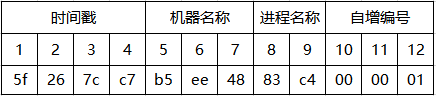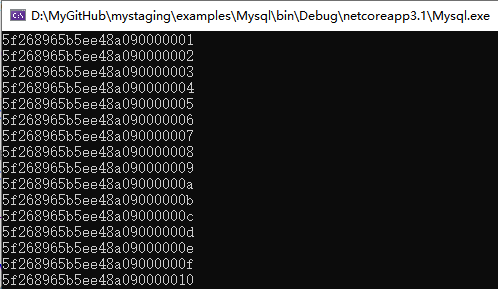后台-插件-广告管理-内容页广告位一(手机)

# .NET Core中反解ObjectId2021-04-18 19:22:36ASP.NET人已围观

ObjectId 的数据结构1、Unix时间戳：Unix时间戳以秒为记录单位，即从1970/1/1 00:00:00 开始到当前时间的总秒数。
2、机器名称：记录当前生产Oid的设备号
3、进程编号：当前运行Oid程序的编号
4、自增编号：在当前秒内，每次调用都将自动增长（已实现线程安全）```public class ObjectIdFactory
{
private int increment;
private readonly UTF8Encoding utf8 = new UTF8Encoding(false);
private readonly DateTime unixEpoch = new DateTime(1970, 1, 1, 0, 0, 0, DateTimeKind.Utc);

public ObjectIdFactory()
{
MD5 md5 = MD5.Create();
machineHash = md5.ComputeHash(utf8.GetBytes(Dns.GetHostName()));
pidHex = BitConverter.GetBytes(Process.GetCurrentProcess().Id);
Array.Reverse(pidHex);
}

/// <summary>
/// 产生一个新的 24 位唯一编号
/// </summary>
/// <returns></returns>
public ObjectId NewId()
{
int copyIdx = 0;
byte[] hex = new byte;
byte[] time = BitConverter.GetBytes(GetTimestamp());
Array.Reverse(time);
Array.Copy(time, 0, hex, copyIdx, 4);
copyIdx += 4;

Array.Copy(machineHash, 0, hex, copyIdx, 3);
copyIdx += 3;

Array.Copy(pidHex, 2, hex, copyIdx, 2);
copyIdx += 2;

byte[] inc = BitConverter.GetBytes(GetIncrement());
Array.Reverse(inc);
Array.Copy(inc, 1, hex, copyIdx, 3);

return new ObjectId(hex);
}

private int GetIncrement() => System.Threading.Interlocked.Increment(ref increment);
private int GetTimestamp() => Convert.ToInt32(Math.Floor((DateTime.UtcNow - unixEpoch).TotalSeconds));
}```

ObjectIdFactory 的内部实现非常的简单，但是也是整个 Oid 程序的核心，在构造函数中获取机器名称和进程编号以备后续生产使用，在核心方法 NewId 中，依次将 Timestamp、machineHash、pidHex、increment 写入数组中，最后调用 new ObjectId(hex) 返回生产好的 Oid。

```类 ObjectId 的代码实现
public class ObjectId
{
private readonly static ObjectIdFactory factory = new ObjectIdFactory();

public ObjectId(byte[] hexData)
{
this.Hex = hexData;
ReverseHex();
}

public override string ToString()
{
if (Hex == null)
Hex = new byte;
StringBuilder hexText = new StringBuilder();
for (int i = 0; i < this.Hex.Length; i++)
{
hexText.Append(this.Hex[i].ToString("x2"));
}
return hexText.ToString();
}

public override int GetHashCode() => ToString().GetHashCode();

public ObjectId(string value)
{
if (string.IsNullOrEmpty(value)) throw new ArgumentNullException("value");
if (value.Length != 24) throw new ArgumentOutOfRangeException("value should be 24 characters");
Hex = new byte;
for (int i = 0; i < value.Length; i += 2)
{
try
{
Hex[i / 2] = Convert.ToByte(value.Substring(i, 2), 16);
}
catch
{
Hex[i / 2] = 0;
}
}
ReverseHex();
}

private void ReverseHex()
{
int copyIdx = 0;
byte[] time = new byte;
Array.Copy(Hex, copyIdx, time, 0, 4);
Array.Reverse(time);
this.Timestamp = BitConverter.ToInt32(time, 0);
copyIdx += 4;
byte[] mid = new byte;
Array.Copy(Hex, copyIdx, mid, 0, 3);
this.Machine = BitConverter.ToInt32(mid, 0);
copyIdx += 3;
byte[] pids = new byte;
Array.Copy(Hex, copyIdx, pids, 0, 2);
Array.Reverse(pids);
this.ProcessId = BitConverter.ToInt32(pids, 0);
copyIdx += 2;
byte[] inc = new byte;
Array.Copy(Hex, copyIdx, inc, 0, 3);
Array.Reverse(inc);
this.Increment = BitConverter.ToInt32(inc, 0);
}

public static ObjectId NewId() => factory.NewId();

public int CompareTo(ObjectId other)
{
if (other is null)
return 1;
for (int i = 0; i < Hex.Length; i++)
{
if (Hex[i] < other.Hex[i])
return -1;
else if (Hex[i] > other.Hex[i])
return 1;
}
return 0;
}

public bool Equals(ObjectId other) => CompareTo(other) == 0;
public static bool operator <(ObjectId a, ObjectId b) => a.CompareTo(b) < 0;
public static bool operator <=(ObjectId a, ObjectId b) => a.CompareTo(b) <= 0;
public static bool operator ==(ObjectId a, ObjectId b) => a.Equals(b);
public override bool Equals(object obj) => base.Equals(obj);
public static bool operator !=(ObjectId a, ObjectId b) => !(a == b);
public static bool operator >=(ObjectId a, ObjectId b) => a.CompareTo(b) >= 0;
public static bool operator >(ObjectId a, ObjectId b) => a.CompareTo(b) > 0;
public static implicit operator string(ObjectId objectId) => objectId.ToString();
public static implicit operator ObjectId(string objectId) => new ObjectId(objectId);
public static ObjectId Empty { get { return new ObjectId("000000000000000000000000"); } }
public byte[] Hex { get; private set; }
public int Timestamp { get; private set; }
public int Machine { get; private set; }
public int ProcessId { get; private set; }
public int Increment { get; private set; }
}```

ObjectId 的代码量看起来稍微多一些，但是实际上，核心的实现方法就只有 ReverseHex() 方法，该方法在内部反向了 ObjectIdFactory.NewId() 的过程，使得调用者可以通过调用 ObjectId.Timestamp 等公开属性反向追溯 Oid 的生产过程。

NewId

```for (int i = 0; i < 100; i++)
{
var oid = ObjectId.NewId();
Console.WriteLine(oid);
}``````var sourceId = ObjectId.NewId();
var reverseId = new ObjectId(sourceId);``````var sourceId = ObjectId.NewId();

// 转换为 string
var stringId = sourceId;
string userId= ObjectId.NewId();

// 转换为 ObjectId
ObjectId id = stringId;```

Tags：.net   core   ObjectId

 后台-插件-广告管理-内容页广告位二(手机)
 后台-插件-广告管理-内容页广告位三(手机)
 后台-插件-广告管理-内容页广告位四(手机)

## 文章评论

 留言与评论（共有 0 条评论）

 验证码：